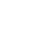Published on

# 8 Must Know JavaScript Array Methods | JavaScript Array operationsAuthors
•Name
Gyanendra Kumar Knojiya
@gyanknojiya

We are going to talk about top JavaScript Array methods that you should know. By using these methods, you can speed up your development.

## 1. Filter

Here we have an array of items-

const list = [
{ name: 'book', price: 2 },
{ name: 'book', price: 2 },
{ name: 'pen', price: 12 },
{ name: 'ink', price: 14 },
{ name: 'rubber', price: 6 },
{ name: 'pencil', price: 4 },
{ name: 'notebook', price: 3 },
{ name: 'camera', price: 20 },
]


Now, we want to filter items that have a price less than 10. To do that, we can filter method-

const filterList = list.filter((item) => item.price < 10)[ // Output
({ name: 'book', price: 2 },
{ name: 'book', price: 2 },
{ name: 'rubber', price: 6 },
{ name: 'pencil', price: 4 },
{ name: 'notebook', price: 3 })
]


## 2. Map

By using this method, we can loop through all array elements and can create a new array. For example, if we want to create an array of items name only, then we can use the map.

const newArray = list.map((item) => item.name)

//Output
;['book', 'book', 'pen', 'ink', 'rubber', 'pencil', 'notebook', 'camera']


## 3. Find

By using the find method, we can find a single item in the array. To find an item, we add a true or false statement and it will return the first item where the condition is true.

const findItem = list.find((item) => item.name === 'camera')

//Output
{name: 'camera', price: 20}


## 4. forEach

It is like for loop in javaScript, but it does not return an array. Just we can loop through all elements in the array.

list.forEach((item) => console.log(item.name))

//Output
'book'
'book'
'pen'
'ink'
'rubber'
'pencil'
'notebook'
'camera'



## 5. Some

Some functions return true or false when a condition is satisfied. It loops through all elements until it finds the condition. If find the condition, it will return true otherwise false.

const hasCamera = list.some((item) => item.name === 'camera')

//Output
true


## 6. Every

It is similar to some but instead of checking one item, it will check every item. If anyone's condition failed, it will return false.

const hasMoreLess20 = list.every((item) => item.price < 20)
//Output
false

const hasMoreLess21 = list.every((item) => item.price < 21)
//Output
true


## 7. Reduce

Reduce loops through all array elements and give an accumulated result.

Syntax: array.reduce( function(total, currentValue), initialValue )

Here, we want to calculate the total price of all items in the array-

const total = list.reduce((total, item) => total + item.price, 0)

//Output
63


## 8. Includes

It will check whether the element is present in the array or not. It takes a single argument as input and returns true or false.

const numbers = [2, 5, 8, 9, 8, 6]


Here we are checking if 9 is in the array or not-

const isNine = numbers.includes(9)

//Output
true


## Array.at

It will return an element at given index.

const numbers = [2, 5, 8, 9, 8, 6]


If we want element at index 3 then

numbers.at(3)
// 9


we can use negative index to get element form last. Negative index will start from -1.

numbers.at(-2)
// 8